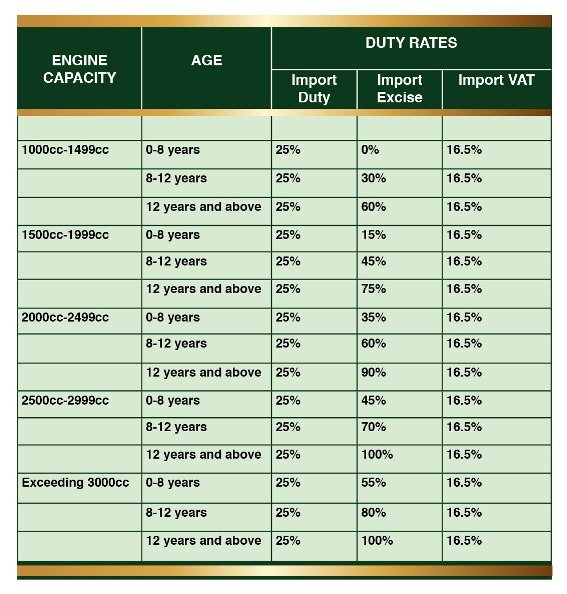# VARIATION OF IMPORT EXCISE RATES ON SALOONS/STATION WAGONS

Many people import saloons or station wagons but do not know that excise rates for such vehicles vary depending on the year the vehicle was manufactured and the engine size. Below is, therefore, a table that illustrates how import excise rates apply for saloons and station wagons for different years of make and engine capacity.

Assume taxpayer X has imported a saloon which is less than eight years old with an engine size of 1500 cc. Taxpayer Y has also imported a similar saloon with an engine size the same as X but is more than twelve years old. Value for Duty Purposes will include the Cost (FOB) + Insurance + Freight value + Port charges) and would be calculated cumulatively as follows:

X.   Where Value for Duty Purposes   is \$5, 714, for example, and the exchange rate is \$1 to K350, the total Customs value would be \$5, 714 x 350=K2, 000, 000.

Duty calculation:

1. Import duty                  = 25% x K2, 000, 000                                     =K500, 000
2. Import excise              =0% x (K2, 000, 000 + K500, 000)                 =K0.00
3. Import VAT                 =16.5% x (K2, 500, 000 + K0.00)                   =K412, 500
4. Total duty payable      =K500, 000 + K0.00 +K412, 500                    =K912, 500

Y.  Where Value for Duty Purposes   is \$3, 714 and the exchange rate is \$1 to K350, the total Customs value would be \$3, 714 x 350=K1, 300, 000.

Duty calculation:

1. Import duty                    = 25% x K1, 300, 000                                                 =K325, 000

2. Import excise                =60% x (K1, 300, 000 + K325, 000)                           =K975, 000

1. Import VAT                 =16.5% x (K1, 300, 000 + K325, 000 + K975, 000)   =K429, 000
2. Total duty payable      = K325, 000 + K975, 000 + K429, 000                       =K1, 729, 000

It is against this background that the Authority would like to advise members of the general public that when importing saloons or station wagons, they should know the year of manufacture and the engine size as the two determine the rate at which excise duty will be computed.